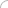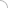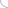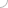# Mathematics interview questions and answers

## Mathematics interview questions and answers

### 1. Three persons A, B and C start traveling from city P at 1:00 a.m., 2:00 a.m. and 3:00 a.m. respectively. All the three reach the point Q simultaneously and continue beyond that point. C reaches the point R first and turns back immediately towards P. Thirty minutes after turning back, he crosses A. If the distance between P and Q is equal to the distance between Q and R, then the time at which all the three reach Q is 2. Ram's age when Shyam was as old as Ram is one-third of the Shyam's present age. When Ram will be as old as Shyam is, Shyam will be 10 years older than Ram will be. Find Shyam's present age (in years). 3. N is a three digit number. 2N equals the sum of the following 4 quantities - the sum of the digits of N, the two digit number formed by the first two digits of N, the two digit number formed by the last two digits of N and 961. The hundreds digit of N is 3 more than its tens digit. Find its tens digit. 4. The sum of a two-digit number and the reversed number is 99. How many such numbers are possible? 5. A went on a tour. He visited a total of 8 cities. In each city he spent Rs.2 less than half the amount he had with him. He spent Rs.100 in the last city he visited. Find the amount he had initially (in Rs.) 6. Ashok's age x years ago was twice his sons age at that time. His age 5x years ago was thrice his sons age at that time. The difference of the present ages of both is 24 years. Find the sum of their present ages. (in years) 7. Two trains enter a tunnel from opposite ends simultaneously. The speeds of the trains are 54 kmph and 72 kmph. After 30 seconds, the trains completely cross each other. After 20 more seconds, the slower train is just out of the tunnel. What is the length of the faster train? (adsbygoogle = window.adsbygoogle || []).push({}); Write your comment - Share Knowledge and Experience (adsbygoogle = window.adsbygoogle || []).push({}); (adsbygoogle = window.adsbygoogle || []).push({});Interview questionsLatest MCQs » General awareness - Banking » ASP.NET » PL/SQL » Mechanical Engineering » IAS Prelims GS » Java » Programming Language » Electrical Engineering » English » C++ » Software Engineering » Electronic Engineering » Quantitative Aptitude » Oracle » English » Finance Home | About us | Sitemap | Contact us | We are hiring © Copyright 2016. All Rights Reserved. Terms of use  |  Follow us on Facebook!